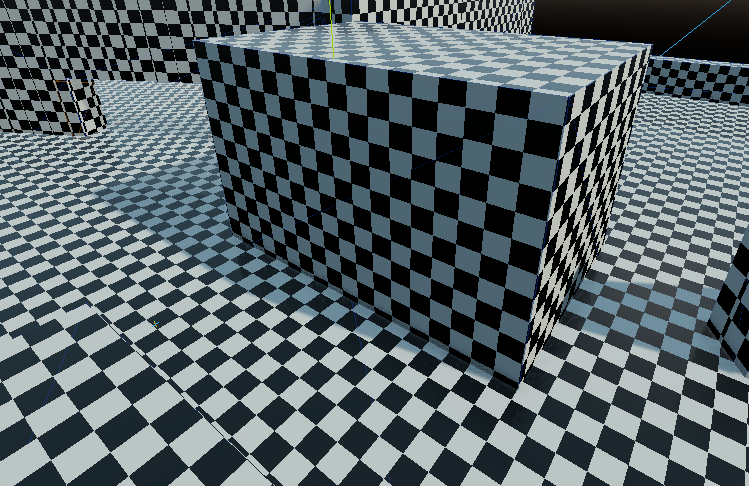# world coordinates grid b&w shader

This shader displays a grid on every surface aligned with the unit vectors, it displays a jagged grid otherwise. This shader is useful for prototyping levels, especially when working with CSGs.

``````shader_type spatial;
varying vec3 world_position;

void vertex() {
world_position = VERTEX;
}

const float gridSize = 3.5;

void fragment(){
vec3 pos = world_position;
pos /= gridSize;
pos += gridSize * 20.0;
//to offset a bug that appears when one of the coordinate is close to 0
//can be tweaked if the bug is visible
pos.y += 1.0*float(fract(float(int(pos.x*2.0))/2.0));
pos.z += float(fract(float(int(pos.y*2.0))/2.0));
vec3 col = vec3(fract(float(int(pos.z*2.0))/2.0));
ROUGHNESS = col.x/2.0 + 0.2;
ALBEDO = col;
}``````
###### Tags
black and white, grid, prototyping, Spatial, world coordinatesThe shader code and all code snippets in this post are under CC0 license and can be used freely without the author's permission. Images and videos, and assets depicted in those, do not fall under this license. For more info, see our License terms.

### 3D Pixel art outline & highlight Shader (Post-processing/object)

Subscribe
Notify ofInline Feedbacks2 years ago

How can i adjust the grid size? I would like to put it in meters2 years ago

I fixed the Zero problem, added color and lines:Belzecue
1 month ago
```	vec3 pos = world_position;
pos /= gridSize;
pos += gridSize * 20.0;
```

This can be moved into the vertex function, because it never changes in fragment() per vertex, so may as well only calculate it per vertex instead of per pixel.

e.g.

```void vertex() {
world_position = VERTEX;
world_position /= gridSize;
world_position += gridSize * 20.0;
}

void fragment(){
vec3 pos = world_position;
...
}
```